# DEFLECTIONS IN DETERMINATE TRUSSESReading time: 1 minute

DEFLECTIONS IN DETERMINATE TRUSSES Trusses comprise of members connected through pin joints. Truss members can thus transmit only axial forces (tensile and compressive forces). The basic difference in the behavior of a truss and a beam is that the truss comprises members that can sustain only axial forces but no bending moment or shear force which are the dominant forces in the beam.The simplest configuration of a truss is a set of interconnected members forming a series of triangles. Here we will discuss the deflections of pin jointed determinate trusses. However, same analysis can be extended to indeterminate trusses as well. Computations of displacements in trusses are required quite often, especially in bridge structures. Displacements in trusses are required not only for the final configurations but also during various stages of erection. Deflections can be due to self-weight, external loading, due to changes in temperatures of the truss members or due to a combination of these effects.

#### Method of computing Deflections in Determinate Trusses:

The most general method of computing displacements in trusses is by the virtual work principle. Since a unit load (virtual load or dummy load that does not exist) is applied to the structure, this method is usually known as dummy load method or unit load method. The displacement at any joint in a truss for a given loading can be determined by applying a unit virtual load in the direction of the required deflection at that joint. The displacement can be computed as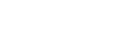…………….. (1)   Where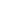= force in member k due to the given loads,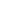= force in member k due to a unit load at joint j,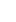= length of member k,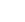= area of member k,=Young’s modulus of member k,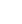= deflection at joint j in the direction of unit load and n= number of truss bars (members) Deflections are also possible due to changes in the length of members due to fabrication errors or changes in temperature. In such cases, applying the principle of virtual work,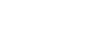………………….2(a)   Where,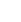= change in length of member k,= change in thermal expansion of member K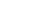= change in temperature. Thus we have,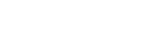……………2(b)   Displacements in trusses are calculated by using Eqn. 1 or 2, as the case may be.